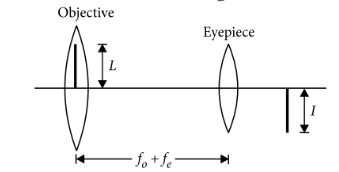Ray optics
Question

# In an astronomical telescope in normal adjustment a straight black line of length L is drawn on inside part of objective lens. The eye-piece forms a real image of this line. The length of this image is I. The magnification of the telescope is

Difficult
Solution

## The situation is shown in the figure.Let ${f}_{0}$ and ${f}_{e}$ be the focal lengths of the objective and eyepiece respectively.For normal adjustment distance of the objective from the eyepiece (tube length) ${f}_{0}$ + ${f}_{e}$Treating the line on the objective as the object and eyepiece as the lens.$\frac{1}{v}=\frac{1}{{f}_{e}}-\frac{1}{{f}_{o}+{f}_{e}}=\frac{{f}_{o}+{f}_{e}-{f}_{e}}{{f}_{e}\left({f}_{o}+{f}_{e}\right)}=\frac{{f}_{o}}{{f}_{e}\left({f}_{o}+{f}_{e}\right)}$$\therefore$The magnification of the telescope in normal adjustment is

Get Instant Solutions